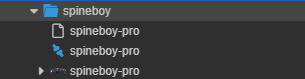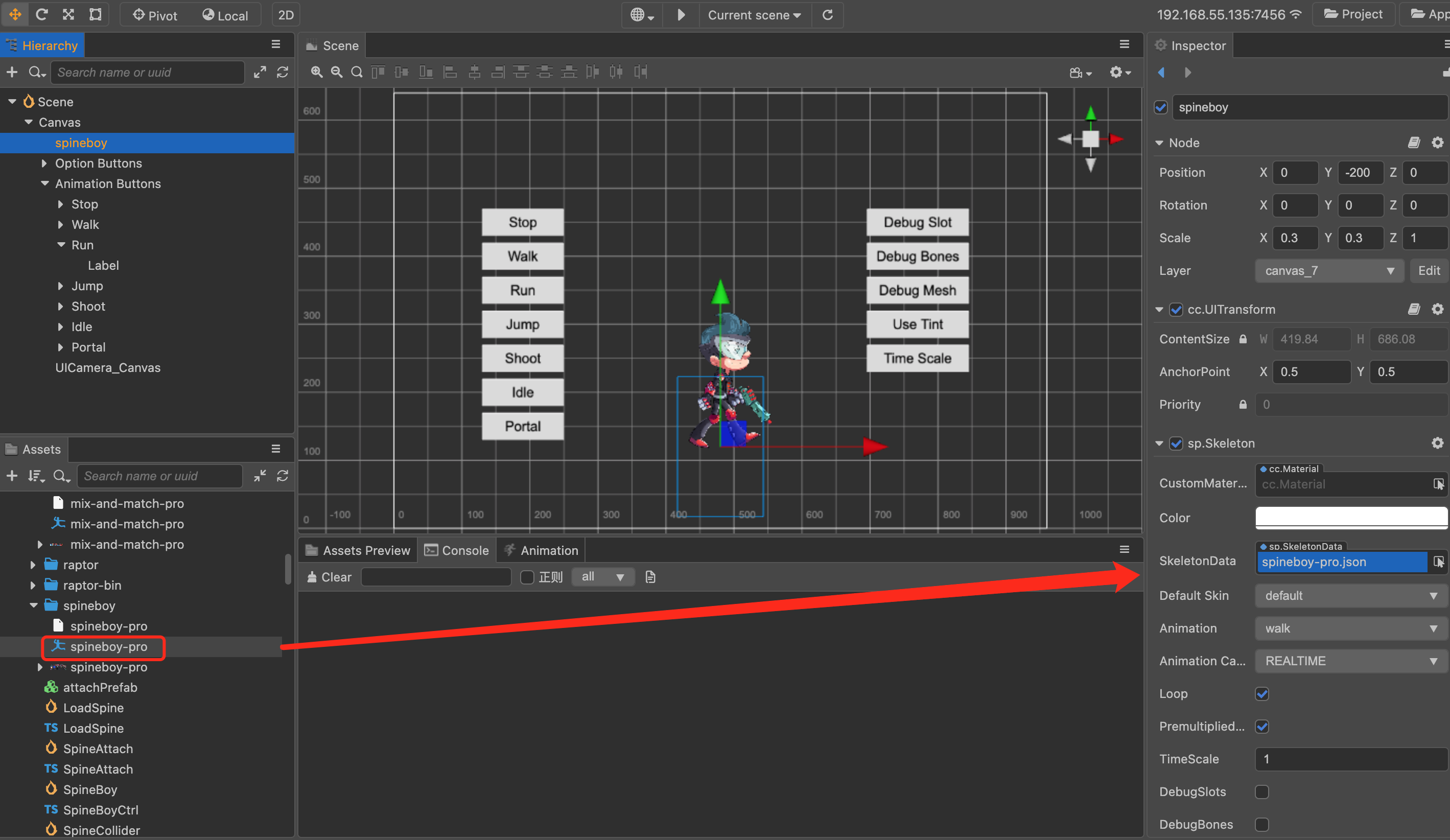# Skeletal Animation Assets (Spine)

Skeletal animation assets in Creator are exported from Spine, which currently supports the JSON and binary data formats.

The supported Spine versions for each Creator version are as follows:

Creator Version Spine Version
v3.0 and above v3.8 (v3.8.75 is not supported on the native platform)
v2.3 and above v3.8
v2.2 v3.7
v2.0.8 to v2.1 v3.6
v2.0.7 and below v2.5

## Import Skeletal Animation Assets

The assets required for skeletal animation are:

• `.json/.skel` skeletal data
• `.png` atlas textures
• `.txt/.atlas` atlas data## Create Skeletal Animation

Drag the skeletal animation asset from the Assets panel to the `SkeletonData` property of the spine component in the Inspector panel.## How to load Spine remotely from a server

### Load the Spine assets in json format

``````let comp = this.getComponent('sp.Skeleton') as sp.Skeleton;

assetManager.loadAny([{ url: atlas, ext: '.txt' }, { url: ske, ext: '.txt' }], (error, assets) => {
assetManager.loadRemote(image, (error, texture: Texture2D) => {
let asset = new sp.SkeletonData();
asset.skeletonJson = assets;
asset.atlasText = assets;
asset.textures = [texture];
asset.textureNames = ['1.png'];
skeleton.skeletonData = asset;
});
});
``````

### Load the Spine assets in binary format

``````let comp = this.getComponent('sp.Skeleton') as sp.Skeleton;

assetManager.loadAny([{ url: atlas, ext: '.txt' }, { url: ske, ext: '.bin' }], (error, assets) => {
assetManager.loadRemote(image, (error, texture: Texture2D) => {
let asset = new sp.SkeletonData();
asset._nativeAsset = assets;
asset.atlasText = assets;
asset.textures = [texture];
asset.textureNames = ['1.png'];
asset._uuid = ske; // Any string can be passed in, but it cannot be empty.
asset._nativeURL = ske; // Pass in a binary path to be used as the 'filePath' parameter when using 'initSkeleton'.
comp.skeletonData = asset;
let ani = comp.setAnimation(0, 'walk', true);
});
});
``````